# MATH 251: Calculus 3, SET8

## 14: Partial Derivatives

### 14.5: The Chain Rule

These problems are done with the CAS. See Hand Solutions for details.

#### 1. [943/4]

We compute a derivative using the Chain Rule (intermediate pieces) then check our work directly (all at once with independent variable).
syms g t x y z real
z = sqrt(1 + x*y), g = [tan(t) atan(t)] % g = [x y] in terms of t
z =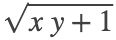g =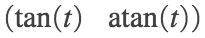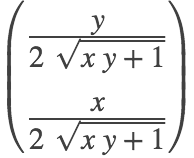Dg =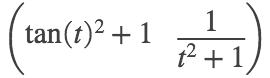dz_dt = dot(grad_z, Dg) % mixed (chaining variables x and y plus independent variable t)
dz_dt =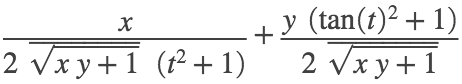dz_dt = subs(dz_dt, [x y], g) % solely in terms of t
dz_dt =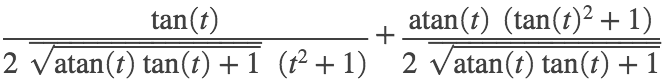% Check
Z = subs(z, [x y], g)
Z =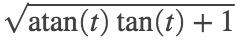dZ_dt = diff(Z,t)
dZ_dt =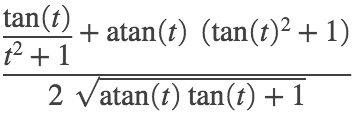#### 2. [943/6]

Again, the Chain Rule allows us to break the problem into smaller simpler pieces, then aggregate results.
syms g t w x y z real
w = log(sqrt(x^2 + y^2 + z^2))
w =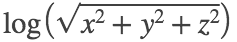g = [sin(t) cos(t) tan(t)], % g = [x y z] in terms of t
g =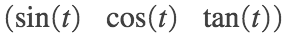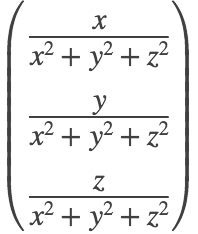Dg =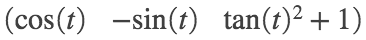dw_dt = dot(grad_w, Dg) % mixed (chaining variables x,y,z plus independent variable t)
dw_dt =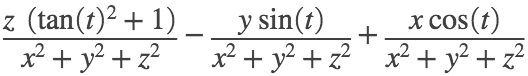dw_dt = simplify(subs(dw_dt, [x y z], g)) % solely in terms of t
dw_dt =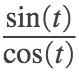% Check
W = subs(w, [x y z], g)
W =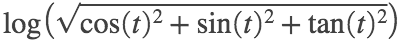dW_dt = simplify(diff(W,t))
dW_dt =#### 3. [943/8]

Here we'll just use the Chain Rule and leave the results in terms of mixed variables.
syms g s t x y real
z = atan(x^2 + y^2), g = [s*log(t) t*exp(s)] % g = [x y] in terms of s and t
z =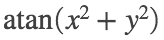g =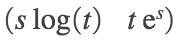grad_z = gradient(z, [x y]), gs = diff(g,s), gt = diff(g,t) % partial derivatives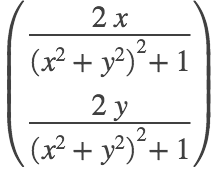gs =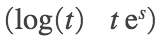gt =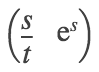zs = dot(grad_z, gs) % mixed
zs =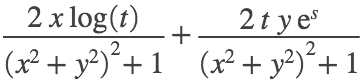zt = dot(grad_z, gt) % mixed
zt =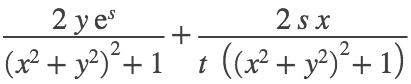#### 4. [944/16]

In this problem we are given a table instead of formulas. See Hand Solutions for the solution written in steps using table values.

#### 5. [944/22]

Here we'll use the Chain Rule with mixed variables then substitute values for these to obtain numerical partial derivative values.
syms g p q r T u v real
T = v / (2*u + v), g = [p*q*sqrt(r) p*sqrt(q)*r] % g = [u v] in terms of p, q, r
T =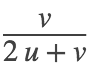g =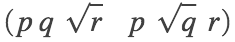grad_T = simplify(gradient(T, [u v])), gp = diff(g,p), gq = diff(g,q), gr = diff(g,r)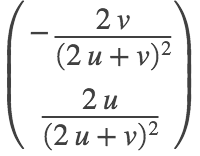gp =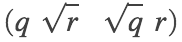gq =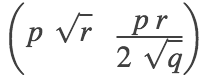gr =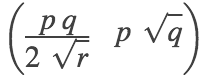Tp =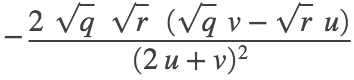Tq =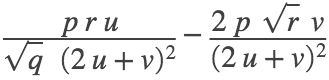Tr =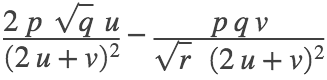uv_num = subs(g, [p q r], [2 1 4])
uv_num =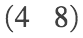Tp_num = subs(Tp, [p q r u v], [2 1 4 4 8])
Tp_num = 0
Tq_num = subs(Tq, [p q r u v], [2 1 4 4 8])
Tq_num =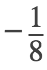Tr_num = subs(Tr, [p q r u v], [2 1 4 4 8])
Tr_num =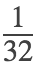#### 6. [944/24]

We proceed the same way as in #5.
syms g u v w x y real
P = sqrt(u^2 + v^2 + w^2), g = [x*exp(y) y*exp(x) exp(x*y)] % g = [u v w] in terms of x, y
P =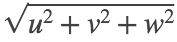g =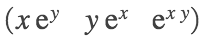grad_P = gradient(P, [u v w]), gx = diff(g,x), gy = diff(g,y)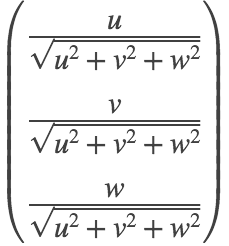gx =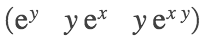gy =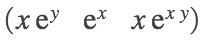Px =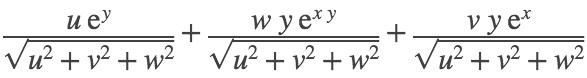Py =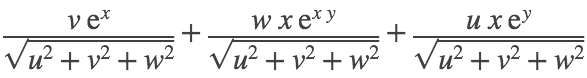uvw_num = subs(g, [x y], [0 2])
uvw_num =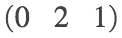Px_num = subs(Px, [u v w x y], [0 2 1 0 2])
Px_num =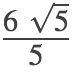Py_num = subs(Py, [u v w x y], [0 2 1 0 2])
Py_num =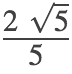#### 7. [944/32]

Here we use the method given on page 943 of the textbook to do implicit differentiation.
syms x y z
F = x^2 - y^2 + z^2 - 2*z - 4 % Let F = LHS - RHS of the equation given, understood to be set 0.
F =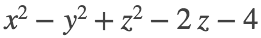zx = simplify( -diff(F,x) / diff(F,z) )
zx =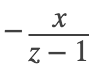zy = simplify( -diff(F,y) / diff(F,z) )
zy =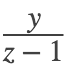#### 8. [945/38]

We use the Chain Rule to compute a rate of change of volume of a cone w.r.t. time.
syms Dh Dr h r V real
V = 1/3 * pi * r^2 * h, g = [h r]
V =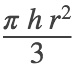g =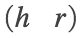grad_V = gradient(V, [h r]), Dg = [Dh Dr] % dg/dt = [dh/dt dr/dt]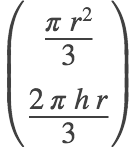Dg =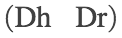dV_dt =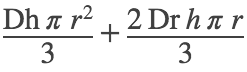dV_dt_num = subs(dV_dt, [Dh Dr h r], [-2.5 1.8 140 120])
dV_dt_num =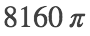format bank
dV_dt_num_appx = double(dV_dt_num) % in cm^3 / s
dV_dt_num_appx = 25635.40
format short

#### 9. [955/42]

We use the Chain Rule to compute a rate of change of production, an economics problem.
syms DK DL K L P real
P = 1.47 * L^0.65 * K^0.35, g = [K L]
P =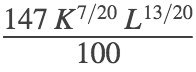g =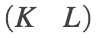grad_P = gradient(P, [K L]), Dg = [DK DL] % dg/dt = [dK/dt dL/dt]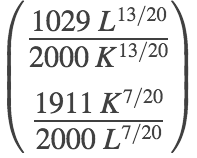Dg =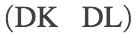dP_dt = dot(grad_P, Dg) % Ignore complex conjugation: the variables are real!
dP_dt =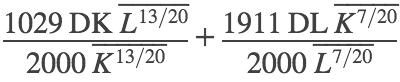dP_dt_num = subs(dP_dt, [DK DL K L], [1/2 -2 8 30])
dP_dt_num =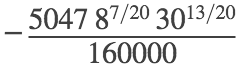dP_dt_num_appx = double(dP_dt_num) % in millions of dollars per year
dP_dt_num_appx = -0.5958
% So production is decreasing at \$596,000 per year.

#### 10. [945/46]

An equation involving partial derivatives is verified.
syms g s t u u_x u_y x y real
g = exp(s)*[cos(t) sin(t)] % g = [x y] in terms of s and t
g =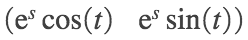grad_u = [u_x u_y], g_s = diff(g,s), g_t = diff(g,t)
grad_u =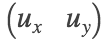g_s =g_t =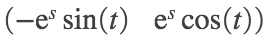u_s =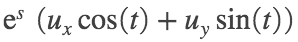u_t =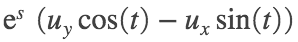LHS =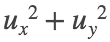RHS =